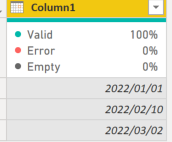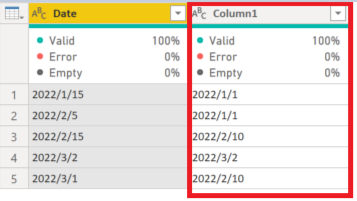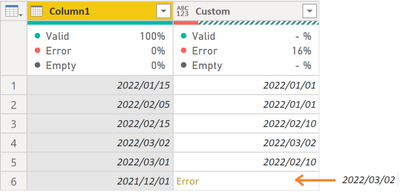cancel
Showing results for
Did you mean:Anonymous
Not applicable

## How to merge two get nearest previous date by using M Code ?

Hi. I am trying to get nearest previous date from other table(Table1) in Power Query not by DAX?

Table1:Expected Result :Any help on how to do this would be highly appreciated.

Thank you.

2 ACCEPTED SOLUTIONSSuper User

See the working here - Open a blank query - Home - Advanced Editor - Remove everything from there and paste the below code to test

This code is for Table2.

``````let
Source = Table.FromRows(Json.Document(Binary.Decompress(Binary.FromText("i45WMjIwMtI1MNQ1NFWK1YFxgQiViyJrDBRB5RoqxcYCAA==", BinaryEncoding.Base64), Compression.Deflate)), let _t = ((type nullable text) meta [Serialized.Text = true]) in type table [Column1 = _t]),
#"Changed Type" = Table.TransformColumnTypes(Source,{{"Column1", type date}}),
BuffList = List.Buffer(Table1[Column1]),
in

Code for Table1

``````let
Source = Table.FromRows(Json.Document(Binary.Decompress(Binary.FromText("i45WMjIwMtI1MAQipVgdGNdI19AAiWsMFFGKjQUA", BinaryEncoding.Base64), Compression.Deflate)), let _t = ((type nullable text) meta [Serialized.Text = true]) in type table [Column1 = _t]),
#"Changed Type" = Table.TransformColumnTypes(Source,{{"Column1", type date}})
in
#"Changed Type"``````Super User

You have done in the right manner. As supplying both input and output makes us give right answer.

You will need to use a try otherwise block

``try [l=List.Transform(BuffList,(x)=>Duration.Days(x-[Column1])),p=BuffList{List.PositionOf(l,List.Last(List.Select(l,(y)=>y<=0)))}][p] otherwise List.Max(BuffList)``

Hence, following code for Table2

``````let
Source = Table.FromRows(Json.Document(Binary.Decompress(Binary.FromText("i45WMjIwMtI1MNQ1NFWK1YFxgQiViyJrDBRB5RrCuEBzjMDcWAA=", BinaryEncoding.Base64), Compression.Deflate)), let _t = ((type nullable text) meta [Serialized.Text = true]) in type table [Column1 = _t]),
#"Changed Type" = Table.TransformColumnTypes(Source,{{"Column1", type date}}),
BuffList = List.Buffer(Table1[Column1]),
in
9 REPLIES 9Super User

See the working here - Open a blank query - Home - Advanced Editor - Remove everything from there and paste the below code to test

This code is for Table2.

``````let
Source = Table.FromRows(Json.Document(Binary.Decompress(Binary.FromText("i45WMjIwMtI1MNQ1NFWK1YFxgQiViyJrDBRB5RoqxcYCAA==", BinaryEncoding.Base64), Compression.Deflate)), let _t = ((type nullable text) meta [Serialized.Text = true]) in type table [Column1 = _t]),
#"Changed Type" = Table.TransformColumnTypes(Source,{{"Column1", type date}}),
BuffList = List.Buffer(Table1[Column1]),
in

Code for Table1

``````let
Source = Table.FromRows(Json.Document(Binary.Decompress(Binary.FromText("i45WMjIwMtI1MAQipVgdGNdI19AAiWsMFFGKjQUA", BinaryEncoding.Base64), Compression.Deflate)), let _t = ((type nullable text) meta [Serialized.Text = true]) in type table [Column1 = _t]),
#"Changed Type" = Table.TransformColumnTypes(Source,{{"Column1", type date}})
in
#"Changed Type"``````Anonymous
Not applicable

@Vijay_A_Verma
I am sorry for asking again.

I got the error for date before Table1.

I would like to get last date(2022/03/02) in Table1 when date is prior to 2022/01/01.

Table1:Expected Result :I know how to replace the error value, but I would like to know how to do it in this formula if possible😣Super User

The way you showed input and output in your original problem statement, in the same way you need to post your requirement.Anonymous
Not applicable

@Vijay_A_Verma
I have edited my post.

Sorry if my understanding is different.

I am not a native speakes so I'm not sure if I understand it properly..😓Super User

You have done in the right manner. As supplying both input and output makes us give right answer.

You will need to use a try otherwise block

``try [l=List.Transform(BuffList,(x)=>Duration.Days(x-[Column1])),p=BuffList{List.PositionOf(l,List.Last(List.Select(l,(y)=>y<=0)))}][p] otherwise List.Max(BuffList)``

Hence, following code for Table2

``````let
Source = Table.FromRows(Json.Document(Binary.Decompress(Binary.FromText("i45WMjIwMtI1MNQ1NFWK1YFxgQiViyJrDBRB5RrCuEBzjMDcWAA=", BinaryEncoding.Base64), Compression.Deflate)), let _t = ((type nullable text) meta [Serialized.Text = true]) in type table [Column1 = _t]),
#"Changed Type" = Table.TransformColumnTypes(Source,{{"Column1", type date}}),
BuffList = List.Buffer(Table1[Column1]),
inAnonymous
Not applicable

Thank you so much!!

It worked perfectly😳Super User

If you make sure BuffList is sorted, then you can simply take the last element that's less than or equal to the date in the current row.

``````let
Source = Table.FromRows(Json.Document(Binary.Decompress(Binary.FromText("i45WMjIwMtI1MNQ1NFWK1YFxgQiViyJrDBRB5RoqxcYCAA==", BinaryEncoding.Base64), Compression.Deflate)), type table [Column1 = text]),
#"Changed Type" = Table.TransformColumnTypes(Source,{{"Column1", type date}}),
BuffList = List.Buffer(List.Sort(Table1[Column1])),
(row) => List.Last(List.Select(BuffList, each _ <= row[Column1])), type date)
inAnonymous
Not applicable

@AlexisOlson

Thank you！I’ll try that too!Anonymous
Not applicable

@Vijay_A_Verma
Thank you for helping me.

I didn't know this solution.
<List.Buffer(Table1[Column1])>

Thank you 😊